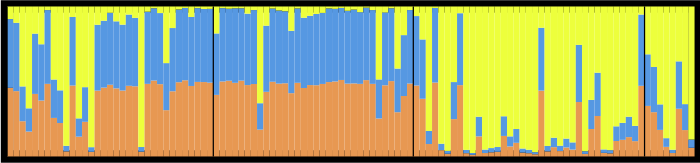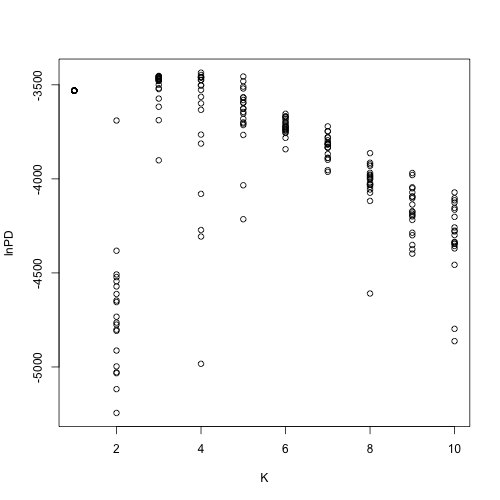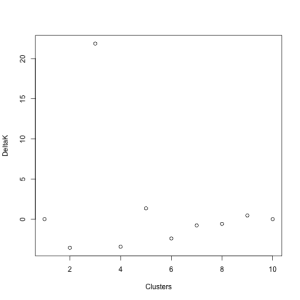# Which K is right for you?STRUCTURE is a program used by scientists who use DNA to infer whether there might be movement of individuals among sampled locales. The program employs a Markov Chain Monte Carlo based Bayesian algorithm to estimate the number of clusters (“K”) and to assign individuals to each cluster based on their genetic makeup at multiple sites (multilocus genotype). You can tell STRUCTURE to assign individuals to any number of clusters you’d like, but you must then choose which is the “best” (i.e. correct/true/most likely to be true) K.

STRUCTURE users often do this by choosing the value of K with the highest value of “Ln P(D)”. STRUCTURE calculates this value by computing the log likelihood of the data for each step of the MCMC, taking their average, and subtracting half their variance. This method is not fail-safe because for multiple runs of each K, the distributions of their values of LnP(D) may have significant overlap (below).Instead, Evanno et al. (2005) proposed a method that is simple and (according to their application to simulated data) more accurate. For each value of K assessed in STRUCTURE, you calculate the mean and standard deviation of the LnP(D) values. Then, calculate the difference between each of these values (L’(K)), calculate the difference between these new values (L’‘(K)), and divide that by the standard deviation. This final value is called DELTAK, and has a strong peak at the “true” number of clusters (K) (below). I made a primitive attempt at calculating this in R, the code for which you can find here. While this takes much longer than doing the same in a spreadsheet program like Excel, it will hopefully speed up the process when I am doing multiple analyses.